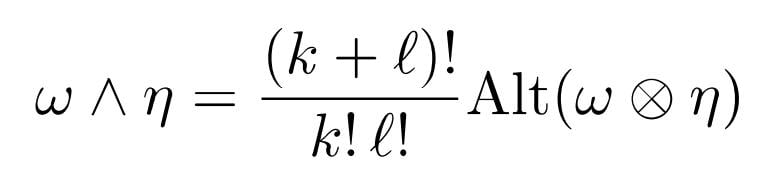# Wedge product

• A
Hello,
we defined the wedge-product as followsAlt is the Alternator and the argument of Alt is the Tensor poduct of one k-form and a l-form (in this order w and eta).
Suppose we have the wedge product of a 0-form (a smooth function) and a l-form , so the following may result:

$$\frac{1}{l!} \sum_{\sigma \in S_k} sgn(\sigma) f \eta(v_{\sigma(1)},...,v_{\sigma(l)}).$$

Does it hold to say that it is equal to $$f*\eta?$$

#### Attachments

Orodruin
Staff Emeritus
Homework Helper
Gold Member
You really should not use ##*## here as it may be mistaken for the hodge operator.

That being said, what do you think? ##f## is clearly independent of the permutation ##\sigma## so you can move it outside the sum. It remains for you to show whether
$$\eta(v_1, \ldots, v_\ell) = \frac{1}{\ell !} \sum_{\sigma \in S_\ell} \operatorname{sgn}(\sigma) \eta(v_{\sigma(1)},\ldots, v_{\sigma(\ell)})$$
or not.

wrobel
In addition, * is a pseudotensor operation while ##\wedge## is a tensor one

Well I weren't sure how to cope with the $$\sigma.$$ My idea was to say that we find for every permutation a counter part, that deviates only by one transposition from our permutation, so to say its form might be $$\sigma \circ \tau,$$ wehere tau is a transposition. By the alternation of the k-form, these parts should vanish. But for the transpositions in the group remain l! possibilities, so that we might get

$$\eta(v_1,...,v_k)-l!\eta(v_1,...,v_k)$$
and if that is not wrong, I don't know how to go on.

fresh_42
Mentor
Well I weren't sure how to cope with the ##\sigma.##
I think this is too complicated.

##\omega \wedge \eta## is a ##(k+l)-##form, so we have to define ##(\omega\wedge\eta)\,(v_1,\ldots,v_{k+l})##. The formula for the alternator is
$$Alt(\omega \wedge \eta)=\dfrac{1}{(k+l)!}\sum_{\sigma\in S_{k+l}}\operatorname{sgn}(\sigma)\,(\omega \wedge \eta)(v_{\sigma(1)},\ldots ,v_{ \sigma(k+l) } )$$
so we have to explain
\begin{align*} (\omega\wedge\eta)\,(v_1,\ldots,v_{k+l})&=\dfrac{1}{k!\,l!}\sum_{\sigma\in S_{k+l}}\operatorname{sgn}(\sigma)(\omega \wedge \eta)\,(v_{\sigma(1)},\ldots ,v_{ \sigma(k+l) } )\\&=\sum_{\sigma\in S_{k+l}}\operatorname{sgn}(\sigma)\,\dfrac{1}{l!}\omega(v_{\sigma(1)},\ldots ,v_{ \sigma(k) }) \wedge \dfrac{1}{k!}\eta(v_{\sigma(k+1)},\ldots ,v_{ \sigma(k+l) }) \end{align*}
This defines a multilinear, associative, anti-commutative and graded multiplication on ##\Lambda (V) = T(V)/\langle v\otimes w-w\otimes v\rangle##.

The role of ##\sigma## is actually only the role of ##\operatorname{sgn}(\sigma)##, which simply is a count of the number of mismatches in the natural order of ##1,\ldots ,k+l## which makes the entire product alternating. The factors ##\dfrac{1}{l!}\; , \;\dfrac{1}{k!}## can be considered as the amount of permutations which do not have an effect:

Say ##k=2## and ##l=3##. Then for every permutation ##\sigma \in S_5## which affects the order of ##(v_1,v_2)## we have ##3!## identical versions which permute the remaining ##3## indices, which is why we cancel them via division, e.g.
$$\omega(v_1,v_2)\stackrel{(*)}{=}\dfrac{1}{3!}\left(\sum_{\sigma=(345)}\omega(v_{\sigma(1)}+v_{\sigma(2)})+\sum_{\sigma=(354)}\omega(v_{\sigma(1)}+v_{\sigma(2)})+\sum_{\sigma=(34)}\omega(v_{\sigma(1)}+v_{\sigma(2)})+\sum_{\sigma=(35)}\omega(v_{\sigma(1)}+v_{\sigma(2)})+\sum_{\sigma=(45)}\omega(v_{\sigma(1)}+v_{\sigma(2)})+\sum_{\sigma=(1)}\omega(v_{\sigma(1)}+v_{\sigma(2)})\right)$$
##(*) ## The sums aren't necessary here as we only sum over one term, they merely serve as a place to write the permutation ##\sigma##.